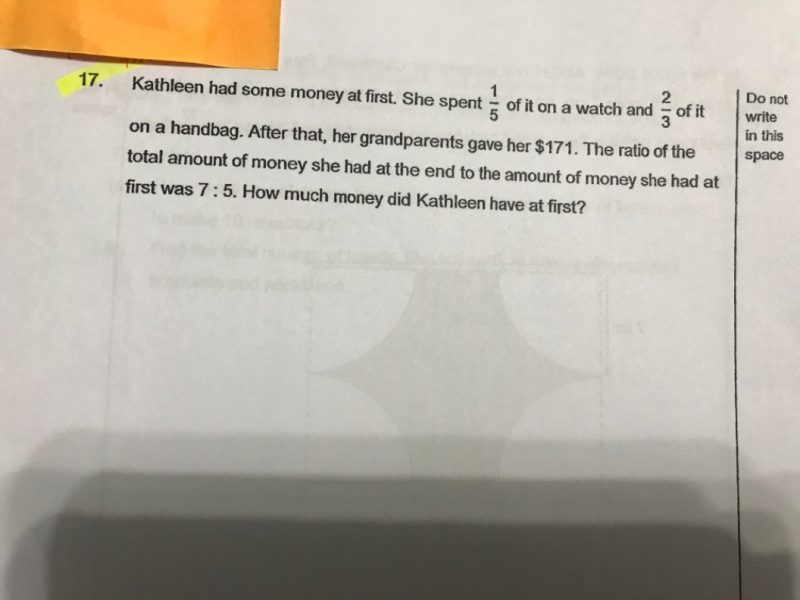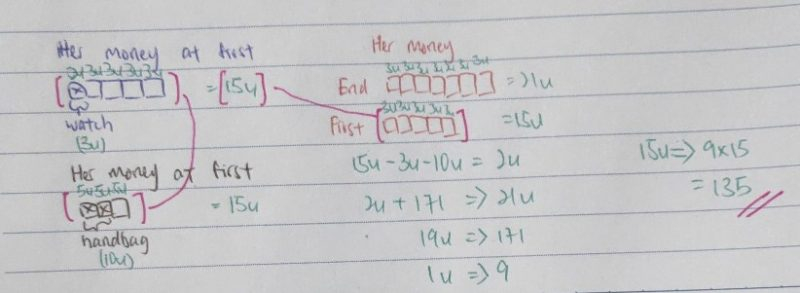QuestionAnyone can  help me to solve this question.

ThanksWebsite

0 Replies 1 Like

Total Spent –>  1/5  + 2/3  = 13/15

Amount left –> 1 – 13/15 = 2/15

Ratio of Amount left : Amount at first  –>  2 : 15

Ratio of Amount at the end : Amount at first –> 7 : 5 = 21 : 15

Amount given by grandparents –> 21 – 2 = 19

19 units = \$171

1 unit = \$171 ÷ 19 = \$9

15 units = 15 x \$9 = \$135